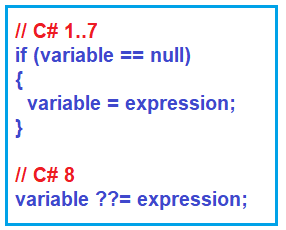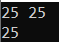# Null-Coalescing Assignment Operator in C#

## Null-Coalescing Assignment Operator in C# 8 with Examples

In this article, I am going to discuss Null-Coalescing Assignment Operator in C# 8 with Examples. Please read our previous article where we discussed Indices and Ranges in C# 8 with Examples. C# 8.0 has introduced a new operator that is known as a Null-Coalescing Assignment Operator (??=).

##### Null-Coalescing Assignment Operator (??=) in C#

C# 8.0 introduces the null-coalescing assignment operator ??=. We can use this ??= operator in C# to assign the value of its right-hand operand to its left-hand operand only if the left-hand operand evaluates to null. That means the null-coalescing assignment operator ??= assigns a variable only if it is null. The syntax is given below.

a ??= b;

Here, a is the left and b is the right operand of the null-coalescing operator ??=. If the value of a is null, then the ??= operator assigns the value of b in a. Or if the value of a is not-null, then it does not evaluate b.

It simplifies a common coding pattern where a variable is assigned a value if it is null. For a better understanding, please have a look at the below diagram. Here, you can observe before C# 8, how we are checking null and assigning a value if it is null and how we can achieve the same in C# 8 using the null-coalescing assignment (??=) operator.##### Points to Remember While working with ??= in C#:
1. The left-hand operand of the ??= operator must be a variable, a property, or an indexer element.
2. It is right-associative.
3. You cannot overload ??= operator.
4. You are allowed to use the ??= operator with reference types and value types.
##### Null-Coalescing Assignment Operator Example in C#:

Let us see an example for a better understanding. The following example is self-explained, so please go through the comment line.

```using System;
using System.Collections.Generic;
namespace Csharp8Features
{
class NullCoalescingAssignment
{
{
List<int>? numbersList = null;
int? number = null;

//numbersList is null, so will create new List<int>() object
numbersList ??= new List<int>();

//As number is null, so it will assign 25 to number and store in the list

//Now number is not null, so it will assign 30 to number,
//so the previous value 25 will store again in the list

Console.WriteLine(string.Join(" ", numbersList));  // output: 25 25
Console.WriteLine(number);  // output:25
}
}
}
```
###### Output:##### Real-Time Use cases of Null-Coalescing Assignment Operator in C#

With the help of Null-Coalescing Assignment ??= Operator in C# 8, we can remove many redundant if-else statements and make our code more readable and understandable. Let us understand this with an example. Here, first, I will show you an example using the if statement, and then I will convert the same example using Null-Coalescing Assignment ??= Operator so that you will get a better idea.

##### Example using If Statements:
```using System;
namespace Csharp8Features
{
class NullCoalescingAssignment
{
{
// C# 1..7
int? Age = null;
if (Age == null)
{
Age = 20;
}
Console.WriteLine(Age);
}
}
}
```
##### Same Example using Null-Coalescing Assignment ??= Operator:
```using System;
namespace Csharp8Features
{
class NullCoalescingAssignment
{